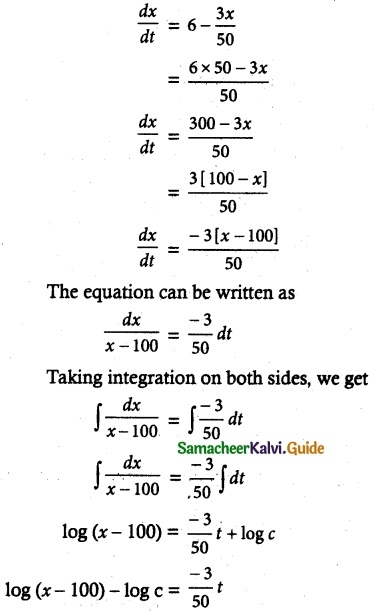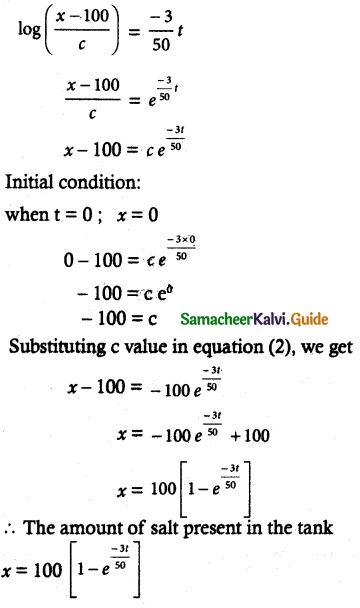Tamilnadu State Board New Syllabus Samacheer Kalvi 12th Maths Guide Pdf Chapter 10 Ordinary Differential Equations Ex 10.8 Textbook Questions and Answers, Notes.

## Tamilnadu Samacheer Kalvi 12th Maths Solutions Chapter 10 Ordinary Differential Equations Ex 10.8

Question 1.
The rate of increase in the number of bacteria in a certain bacteria culture is proportional to the number present. Given that the number triples in 5 hours, find how many bacteria will be present after 10 hours?
Solution:
Let x denote the number of bacteria at time t hours.
Given = $$\frac { dx }{ dt }$$ = kx hence $$\frac { dx }{ x }$$ = kdt
∴ x = C ekt
Suppose x = x0 at time t = 0
x0 = C ek(0) = C e° = C
∴ C = x0
Hence x = x0 ekt
At time 5, x = 3x0
(∵ Number triple in 5 hrs)
∴ Hence 3x0 = x0 e5k
∴ e5k = 3
when t = 10, x = x0 e10k = x0 (e5k
= x0 3² = 9x0
∴ After 10 hours, the number of bacteria as 9 times the original number of bacteria.Question 2.
Find the population of a city at any time t, given that the rate of increase of population is proportional to the population at that instant and that in a period of 40 years, the population increased from 3,00,000 to 4,00,000.
Solution:
Let P denote the population of a city
Given $$\frac { dP }{ dt }$$ = kP
This equation can be written as
$$\frac { dP }{ P }$$ = kdt
Taking integration on both sides, we get
∫ $$\frac { dP }{ P }$$ = k∫ dt
log P = kt + log c
log P – log c = kt
log ($$\frac { P }{ c }$$) = kt
$$\frac { P }{ c }$$ = ekt
P = cekt …….. (1)
Initial condition:
Given when t = 0 ; P = 3,00,000
Equation (1) becomes,
3.0. 000 = cek(0) = ce°
3,00,000 = c [∵ e° = 1]
∴ (1) ⇒ P = 3,00,000 ekt ………. (2)
Again when t = 40; P = 4,00,000
Equation (2) becomes,
4,00,000 = 3,00,000 e40k
$$\frac { 4,00,000 }{ 3,00,000 }$$ = e40k
$$\frac { 4 }{ 3 }$$ = e40k
Taking log,
log($$\frac { 4 }{ 3 }$$) = 40k
k = $$\frac { 1 }{ 40 }$$ log $$\frac { 4 }{ 3 }$$
k = log($$\frac { 4 }{ 3 }$$)$$\frac { 1 }{ 40 }$$ [∵ n log m = log mⁿ]
Substituting k values in equation (2), we get
P = 3,00,000 elog($$\frac { 4 }{ 3 }$$)$$\frac { 1 }{ 40 }$$
P = 3,00,000 ($$\frac { 4 }{ 3 }$$)$$\frac { 1 }{ 40 }$$ [∵ elog a x = ax]Question 3.
The equation of electromotive force for an electric circuit containing resistance and self-inductance is E = Ri + L$$\frac { di }{ dt }$$, where E is the electromotive force is given to the circuit, R the resistance and L, the coefficient of induction. Find the current i at time t when E = 0.
Solution:This is a linear differential equation.
Integrating factor I.F = e∫ $$\frac { R }{ L }$$dt = e$$\frac { R }{ L }$$t
Its solution is given by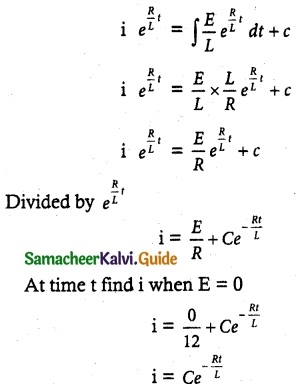Question 4.
The engine of a motorboat moving at 10 m/s is shut off. Given that the retardation at any subsequent time (after shutting off the engine) equal to the velocity at that time. Find the velocity after 2 seconds of switching off the engine.
Solution:
Let v be the velocity
Given the engine of a motorboat moving 10 m/s.
After that the engine is shut off then the acceleration is negative.
So it be $$\frac { -dv }{ dt }$$
i.e., $$\frac { dv}{ dt}$$ = -v
The equation can be written as taking integration on both sides, we get
$$\frac { dv}{ dt}$$ = -dt
∫ $$\frac { dv}{ v }$$ = ∫-dt
log v = -t + log c
log v – log c = -t
log ($$\frac { v }{ c }$$) = -t
$$\frac {v}{c}$$ = e-t
v = ce-t ……….. (1)
Initial condition
Given that when t = 0, v = 10 m/sec i
substituting in equation (1), we get !
10 = ce-0
10 = ce°
10 = c
∴ c = 10
(1) ⇒ v = 10e-t
when t = 2 find v
v = 10 e-2
v = $$\frac {10}{e^2}$$
The velocity after 2 seconds is $$\frac {10}{e^2}$$
i.e., v = $$\frac {10}{e^2}$$Question 5.
Suppose a person deposits 10,000 Indian rupees in a bank account at the rate of 5% per annum compounded continuously. How much money will be in his bank account 18 months later?
Solution:
Let P be the principal amount
Given Rate of interest = 5% per annum.
∴ $$\frac {dp}{dt}$$ = p($$\frac {5}{100}$$) = 0.05P
The equation can be written as,
$$\frac {dP}{P}$$ = 0.05 dt
Taking Integration on both sides, we get
∫$$\frac {dP}{P}$$ = 0.051 ∫dt
log P = 0.05 t + log c
log P – log C = 0.05t
log ($$\frac {P}{C}$$) = 0.05 t
$$\frac {P}{C}$$ = e0.05t
P = C e0.05t ………. (1)
Initial condition:
Given when t = 0; P = 10,000
Substituting these values in equation (1), we get
P = C e0.05t
10,000 = C e0.05 (0)
10,000 = C e°
C = 10,000
∴ Substituting the C value in equation (1), we get
P = 10,000 e0.05t ……. (2)
When t= 18 months = 1$$\frac {1}{2}$$yr =3/2 years, we get
(2) ⇒ P = 10,000 e0.05 (3/2)
P = 10,000 e0.075
The amount in a bank account be
P = 10,000 e0.075Question 6.
Assume that the rate at which radioactive nuclei decay is proportional to the number of such nuclei that are present in a given sample. In a certain sample, 10% of the original number of radioactive nuclei have undergone disintegration in a period of 100 years. What percentage of the original radioactive nuclei will remain after 1000 years?
Solution:
Let N be the sample radioactive nuclei at any time t & N0 be the radioactive nuclei at the initial time.
Given $$\frac {dN}{dt}$$ = -kN
Where k > 0 is a constant
The equation can be written as
$$\frac {dN}{N}$$ = -kdt
Taking integration on both sides, we get
∫$$\frac {dN}{N}$$ = ∫-kdt
log N = -kt + log C
log N – log C = -kt
log($$\frac {N}{C}$$) = -kt
$$\frac {N}{C}$$ = e-kt
N = Ce-kt ……… (1)
Initial condition:
when t = 0 we have N = N0
N0 = Ce-k(0)
N0 = Ce0
N0 = C
Substituting C value in equation (1), we get ;
N = N0 e-kt ……… (2)
Given: In a certain sample 10% of the original number of radioactive nuclei have undergone disintegration in a period of 100 years be when t = p0Equation (2) becomes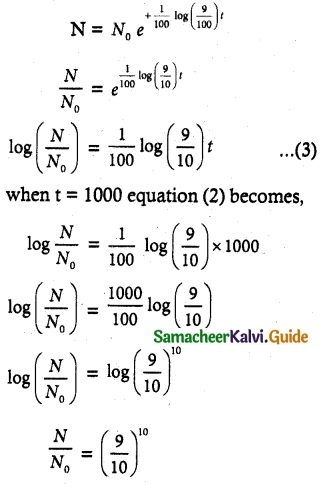The percentage of the original radioactive nuclei of remain after 1000 years is
$$\frac {N}{N_0}$$ × 100 = ($$\frac {9}{10}$$)10 × 100 = $$\frac {9^{10}}{10^{10}}$$ × 10²
= $$\frac {9^{10}}{10^{8}}$$ %Question 7.
Water at temperature 100°C cools in 10 minutes to 80° C at a room temperature of 25°C. Find
(i) The temperature of the water after 20 minutes
(ii) The time when the temperature is 40° C
[log, $$\frac {11}{15}$$ = -0.3101; loge 5 = 1.6094]
Solution:
We apply “Newton’s law of cooling” while states that the rate of decrease of the temperature of a body is proportional to the difference between the temperature of the body and that of the medium.
$$\frac {dT}{dt}$$ = -k(T – T0)
Where T is the temperature of the body at time t & T0 the constant temperature of the medium.
Thus $$\frac {dT}{dt}$$ = -k(T – 25) or
$$\frac {dT}{T-25}$$ = -kdt
On Integrating equation (1), we get
∫$$\frac {dT}{T-25}$$ = ∫-kdt
log (T – 25) = -kt + c1
Now T = 100, t = 0
log (100 – 25) = -k (0) + c1
∴ log 75 = c1
Substituting c1 value in equation (1), we get
log (T – 25) = -kt + log 75
∵ log m – log n = log m/n
log $$\frac {T-25}{75}$$ = kt ……. (2)
Also T = 80 when t = 10 minutes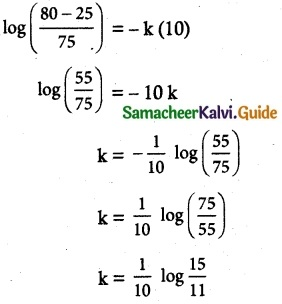Substituting k value in equation (2), we get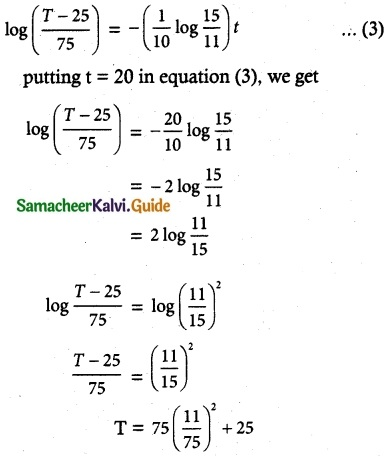T = 75 (0.5378) + 25
T = 40.33 + 25
T = 65.33°CQuestion 8.
At 10.00 A.M. a woman took a cup of hot instant coffee from her microwave oven and placed it on a nearby kitchen counter to cool. At this instant, the temperature of the coffee was 180° F and 10 minutes later it was 160° F. Assume that the constant temperature of the kitchen was 70° F.
(i) What was the temperature of the coffee at 10.15 AM?
The woman likes to drink coffee when its temperature is between 130° F and 140° F. Between what times should she have drunk the coffee?
Solution:
Let T be the temperature of a coffee at time t.
Tk be the temperature of the kitchen
By Newton’s law of cooling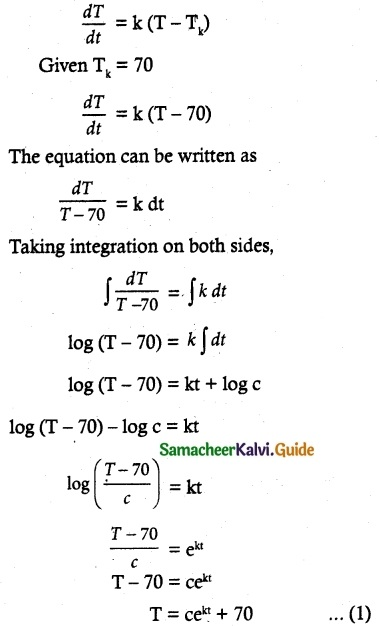Initial condition:
when t = 0; T = 180°F
180 = cek(0) + 70
180 = ce° + 70
180 – 70 = c
∴ c = 110°
Substituting c value in equation (1), we get
T = ce+kt + 70
T = 100 ekt + 70 …….. (2)
Second condition:
when t = 10, T = 160
(2) ⇒ 160 = 110 e10k + 70
160 – 70= 110 e10k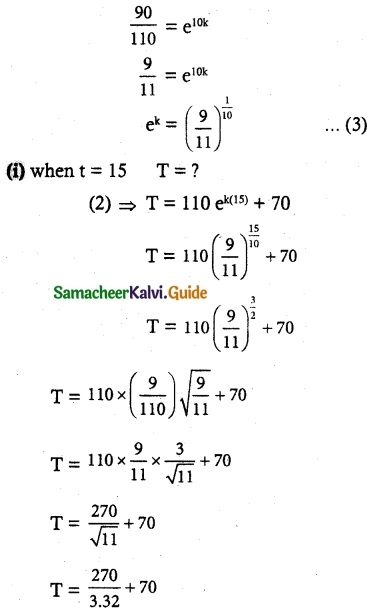T = 81.33 + 70
T = 151.3 F
∴ The temperature of the coffee at 10.15 A.M is 151.3° F

(ii) Woman’s like to drink a coffee between 130°F and 140°F.
(a) when T = 130°F
(2) ⇒ T = 110 ekt + 70
130 – 70 = 110 ekt
$$\frac { 60 }{ 110 }$$
$$\frac { 6 }{ 11 }$$ = ekt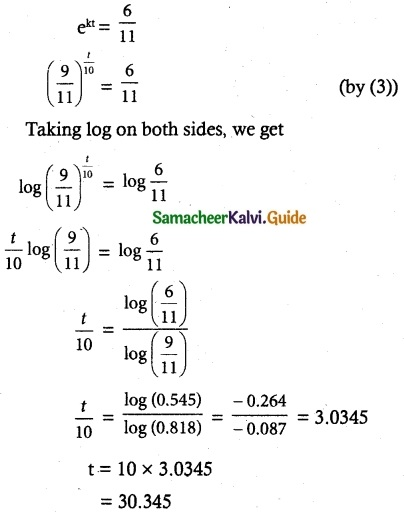b) When T = 140°
(2) ⇒ T = 110ekt + 70
160 – 70 = 110 ektt = 22.6 min
She drinks coffee between 10.22 & 10.30 approximately.Question 9.
A pot of boiling water at 100° C is removed from a stove at time t = 0 and left to cool in the kitchen. After 5 minutes, the water temperature has decreased to 80° C and another 5 minutes later it has dropped to 65° C. Determine the temperature of the kitchen.
Solution:
Let T be the temperature of the boiling water.
Tm is the temperature of the kitchen.
By Newton’s law of cooling, we get
$$\frac { dT }{ dt }$$ = k (T – Tm)
The equation can be written as
Taking Integration on both sides, we get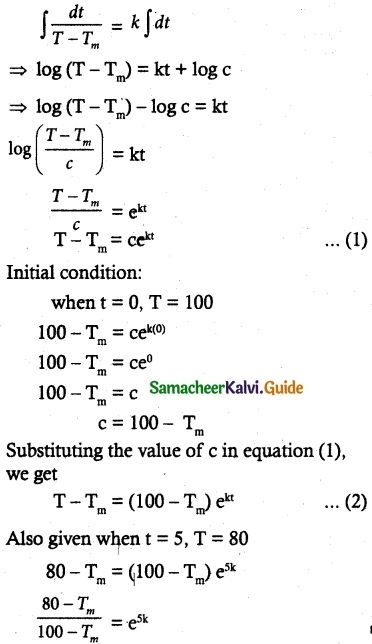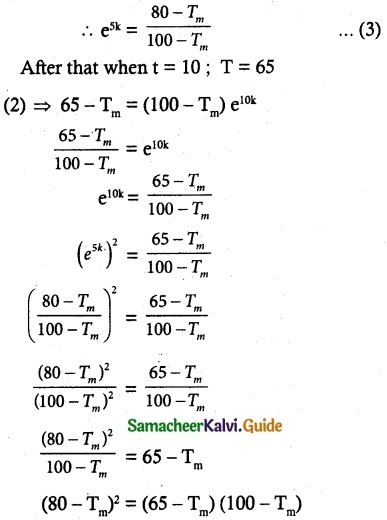(a – b)² = a² + b² – 2ab
a = 80 b = Tm
6400 + T$$_{m}^{2}$$ – 160 Tm = 6500 – 100 Tm – 65 Tm + T$$_{m}^{2}$$
6400 – 6500 = 160 Tm – 165 Tm
– 100 = – 5Tm
Tm = $$\frac { 100 }{ 5 }$$
Tm = 20°C
Hence the temperature of the kitchen be 20° CQuestion 10.
A tank initially contains 50 litres of pure water. Starting at time t = 0 a brine containing 2 grams of dissolved salt per litre flows into the tank at the rate of 3 litres per minute. The mixture is kept uniform by stirring and the well-stirred mixture simultaneously flows out of the tank at the same rate. Find the amount of salt present in the tank at any time t > 0.
Solution:
Let x be the amount of salt in the tank at time t.
∴ $$\frac { dx }{ dt }$$ = inflow rate – outflow rate ……… (1)
Given 2 grams of dissolved salt per litre flows into the tank at the rate of 3 litres per minute.
(i.e) inflow rate contain = 6 gram salt
[∵ for one litre = 2 gram salt]
[for 3 litre = 6 gram salt]
tank contain 50 litres of water
∴ out flow of rate of salt = $$\frac { 3x }{ 50 }$$
substitute inflow rate and outflow rate in equation (1), we get